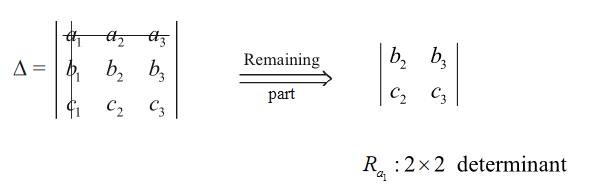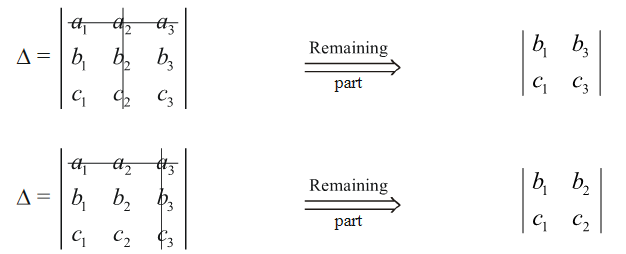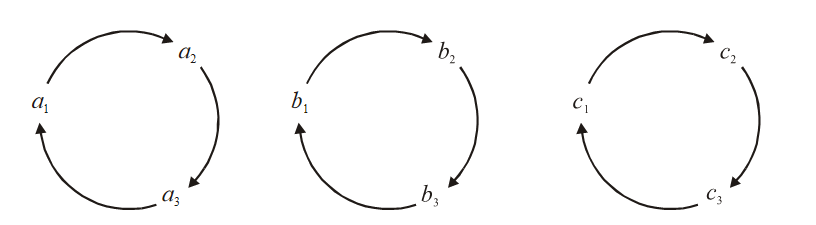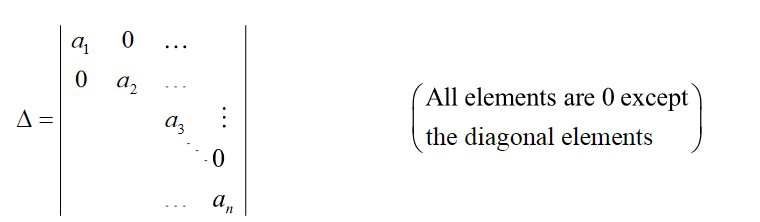# Introduction To Determinants

Go back to  'Determinants and Matrices'

A 2 × 2 determinant is represented by

$\Delta = \left| {\;\begin{array}{*{20}{c}}a&b\\c&d\end{array}\;} \right|$

and its numerical value is $$\Delta = ad - bc.$$

What is the motivation behind defining this quantity? Well, for the moment, think of it as a convenient arrangement of numbers, with a specific rule to calculate its value. If you’ve used determinants in other topics in Math like coordinate geometry or solving simultaneous linear equations, you should be aware of the wide applications of determinants. If not, you should for now focus on what determinants are, and with time, you’ll become familiar with what we can do with them.

Consider a 3 × 3 determinant:

$\Delta = \left| {\;\begin{array}{*{20}{c}}{{a_1}}&{{a_2}}&{{a_3}}\\{{b_1}}&{{b_2}}&{{b_3}}\\{{c_1}}&{{c_2}}&{{c_3}}\end{array}\;} \right|$

Fix your attention on the term a1. If you ignore the row and column passing through a1, the remaining part is a 2 × 2 determinant. Let us call that $${R_{{a_1}}}$$Similarly, we can define $${R_{{a_2}}}\;{\rm{and}}\;{R_{{a_3}}}$$ :In terms of $${R_{{a_1}}},{R_{{a_2}}}\;{\rm{and}}\;{R_{{a_3}}},$$ the value of  $$\Delta$$ is given by:

\begin{align} \Delta =\left| \begin{matrix} {{a}_{1}} & {{a}_{2}} & {{a}_{3}} \\ {{b}_{1}} & {{b}_{2}} & {{b}_{3}} \\ {{c}_{1}} & {{c}_{2}} & {{c}_{3}} \\ \end{matrix} \right| &={{a}_{1}}{{R}_{{{a}_{1}}}}-{{a}_{2}}{{R}_{{{a}_{2}}}}+{{a}_{3}}{{R}_{{{a}_{3}}}} \\ \\ & ={{a}_{1}}\left| \begin{matrix} {{b}_{2}} & {{b}_{3}} \\ {{c}_{2}} & {{c}_{3}} \\\end{matrix} \right|-{{a}_{2}}\left| \begin{matrix} {{b}_{1}} & {{b}_{3}} \\ {{c}_{1}} & {{c}_{3}} \\\end{matrix} \right|+{{a}_{3}}\left| \begin{matrix} {{b}_{1}} & {{b}_{2}} \\ {{c}_{1}} & {{c}_{2}} \\\end{matrix} \right| \\ & \\ & ={{a}_{1}}\left( {{b}_{2}}{{c}_{3}}-{{b}_{3}}{{c}_{2}} \right)-{{a}_{2}}\left( {{b}_{1}}{{c}_{3}}-{{b}_{3}}{{c}_{1}} \right)+{{a}_{3}}\left( {{b}_{1}}{{c}_{2}}-{{b}_{2}}{{c}_{1}} \right) \\ \end{align}

This expansion of $$\Delta$$ is said to be ‘along the 1st row’. We can expand the determinant along any of the rows, and in fact any of the columns too. More on this soon.

Note the ‘cyclicity’ in the expanded version of $$\Delta$$ . Write it as follows:

$\Delta = \left( {{a_1}{b_2}{c_3} + {a_2}{b_3}{c_1} + {a_3}{b_1}{c_2} } \right) - \left( {{a_1}{b_3}{c_2} + {a_2}{b_1}{c_3} + {a_3}{b_2}{c_1} } \right)$

In each bracket, there is a cyclic permutation:This cyclicity is a deliberate property of determinants, making them so useful in elegantly expression long algebraic relations.

To see how a determinant can be expanded generally, along any of its rows or any of its columns, we need to define something called co-factor.

Consider an n × n determinant and denote by $${a_{ij}}$$ that element which is in the $${i^{{\rm{th}}}}$$ row and $${j^{{\rm{th}}}}$$ column. Each $${a_{ij}}$$ will have its corresponding co-factor  $${C_{ij}}$$ which is obtained as follows:

If we ignore the $${i^{{\rm{th}}}}$$ row and  the $${j^{{\rm{th}}}}$$ column, we are left with an  $$\left( {n - 1} \right)\; \times \;\left( {n - 1} \right)$$ determinant. Denote the value of that determinant by $${M_{ij}}.$$ Now $${C_{ij}}$$ will be

${C_{ij}} = {\left( { - 1} \right)^{i + j}}{M_{ij}}$

Note carefully what the $${\left( { - 1} \right)^{i + j}}$$ part will do? For positions where $$i + j$$ is even, $${C_{ij}} = {M_{ij}},$$ while $$i + j$$ being odd will mean that $${C_{ij}} = - {M_{ij}}.$$ For example, for the first row, first column term $$i + j = 2,$$,  so $${C_{ij}} = {M_{ij}}.$$ For the third row, second column term $${C_{ij}} = - {M_{ij}}.$$, In fact ‘+’ and ‘–’ prefixing $${M_{ij}}$$ fall into a straightforward pattern:

$\left| {\;\begin{array}{*{20}{c}} + & - & + & - & \ldots \\ - & + & - & + & \ldots \\ + & - & + & - & \ldots \\ - & + & - & + & \ldots \\ \vdots & \vdots & \vdots & \vdots &{}\end{array}\;} \right|$

To gain a litter practice, let us try a concrete example. Let

$\Delta = \left| {\;\begin{array}{*{20}{c}}3&1&{ - 3}\\2&4&{ - 1}\\3&0&8\end{array}\;} \right|$

What is the co-factor of 4? The position of 4 is (2, 2) so we will not have to put a - sign:

$\text{Co-factor}\ \text{of}\ 4:\left| \ \begin{matrix} 3 & -3 \\ 3 & 8 \\\end{matrix}\ \right|\ =\ 3\times 8-\left( -3\times 3 \right)=33$

What is the co-factor of 0? The position of 0 is (3, 2), so we will have to put a - sign:

$\text{Co-factor}\ \text{of}\ 0:-\left| \ \begin{matrix} 3 & -3 \\ 2 & -1 \\\end{matrix}\ \right|\ =\ -\left( \left( 3x-1 \right)-\left( -3\times 2 \right) \right)=-3$

Let us find co-factor in a  $$4\times 4$$ determinant.

Let us denote the rows as $${{R}_{1}},{{R}_{2}},....,\ {{R}_{n}}$$ and the columns as $${{C}_{1}},{{C}_{2}},....,\ {{C}_{n}}.$$

Expansion along the pth column Rp

The terms in $${{R}_{p}}\ \text{are}\left( \text{say} \right){{a}_{p1}},{{a}_{p2}},....{{a}_{pn}}.$$ They will have corresponding co-factors $${{C}_{p1}},{{C}_{p2}},....,{{C}_{pn}}.\ \ \Delta$$ is given by

$\Delta ={{a}_{p1}}{{C}_{p1}}+{{a}_{p2}}{{C}_{p2}}+...+{{a}_{pn}}{{C}_{pn}}$

That is, multiply each term in the row by its co-factor and add.

Expansion along the qth column Cq

This is exactly the same as expanding along rows. The terms in $${{C}_{q}}$$ will be $${{a}_{1q}},{{a}_{2q}},.....,{{a}_{nq}}$$ and their corresponding co-factors will be $${{C}_{1q}},{{C}_{2q}},...,{{C}_{nq}}$$ , so

$\Delta ={{a}_{1q}}{{C}_{1q}}+{{a}_{2q}}{{C}_{2q}}+...+{{a}_{nq}}{{C}_{nq}}$

Let us expand a 4 × 4 determinant, first along a row and then along a column, and verify that we’ll get the same values.

Example - 1

Let

$\Delta =\left| \ \begin{matrix} 1 & 3 & 2 & -1 \\ 0 & 2 & 4 & 7 \\ 4 & 1 & 3 & 9 \\ 8 & 5 & -2 & 6 \\ \end{matrix}\ \right|$.

Find the co-factors of 6, 7 and 8.

Solution: 6 is at the position $$\left( 4,\ 4 \right)\ \text{so}\ i+j=8.$$ The co-factor of 6 becomes

\begin{align} \left| \ \begin{matrix} 1 & 3 & 2 \\ 0 & 2 & 4 \\ 4 & 1 & 3 \\\end{matrix}\ \right|\ &=\ 1\,\left| \ \begin{matrix} 2 & 4 \\ 1 & 3 \\\end{matrix}\ \right|\ -3\ \left| \ \begin{matrix} 0 & 4 \\ 4 & 3 \\\end{matrix}\ \right|\ +2\left| \ \begin{matrix} 0 & 2 \\ 4 & 1 \\\end{matrix}\ \right| \\ & \\ & =1\times 2-3\times \left( -16 \right)+2\ \times \ \left( -8 \right) \\ & \\ & =34 \\ \end{align}

7 is at the position (2, 4), so $$i+j=6.$$ The co-factor of 7 is

\begin{align} \left| \ \begin{matrix} 1 & 3 & -1 \\ 4 & 1 & 9 \\ 8 & 5 & 6 \\\end{matrix}\ \right|&=\ 1\,\left| \ \begin{matrix} 1 & 9 \\ 5 & 6 \\\end{matrix}\ \right|\ -3\ \left| \ \begin{matrix} 4 & 9 \\ 8 & 6 \\\end{matrix}\ \right|\ +\left( -1 \right)\left| \ \begin{matrix} 4 & 1 \\ 8 & 5 \\\end{matrix}\ \right| \\ & \\ & =1\times \left( -39 \right)-3\times \left( -48 \right)+\left( -1 \right)\times {{i}_{2}} \\ & \\ & =93 \\ \end{align}

For 8, $$i+j=5$$, so its co-factor is

$\left( -1 \right)\ \left| \ \begin{matrix} 3 & 2 & -1 \\ 2 & 4 & 7 \\ 1 & 3 & 9 \\\end{matrix}\ \right|=-65\ \ \ \ \ \ \ \left( \text{verifty} \right)$

Example - 2

Consider the determinant of Example - 1:

$\Delta =\left| \ \begin{matrix} 1 & 3 & 2 & -1 \\ 0 & 2 & 4 & 7 \\ 4 & 1 & 3 & 9 \\ 8 & 5 & -2 & 6 \\\end{matrix}\ \right|$

Expand this along the 2nd row and then along the 3rd column.

Solution: The co-factors of the terms in the 2nd row are

${C_{21}} = - 203 \qquad {C_{22}} = 164 \qquad {C_{23}} = - 93 \qquad {C_{24}} = 103$

Make sure you arrive at these values yourself. Now we have

$\Delta = - \;0\; \times 203 + 2 \times 164 - 4 \times 93 + 7 \times 103 = 677$

Now lets find the co-factors of the terms in the 3rd column:

${C_{13}} = 180 \qquad {C_{23}} = - 93 \qquad {C_{33}} = 161 \qquad {C_{43}} = - 103$

So,

$\Delta = 2 \times 180 - 4 \times 93 + 3 \times 161 - \left( { - 2} \right) \times 103 = 677$

Therefore, the determinant can be expanded equally validly along any row or any column.

As an exercise, expand $$\Delta$$ along the 3rd row and along the 4th column.

From this example, let us note a very interesting fact. The elements in the Ist row are (1, 3, 2, –1) and the co-factors of the elements in the 2nd row are (–203, 164, – 93, 103). Multiply the corresponding numbers and add:

$\left( {1 \times - 203} \right) + \left( {3 \times 164} \right) + \left( {2 \times - 93} \right) + \left( { - 1 \times 103} \right) = 0$

Now, do the same for the elements in the 3rd row, (4, 1, 3, 9), and the 2nd row co-factors:

$\left( {4 \times - 203} \right) + \left( {1 \times 164} \right) + \left( {3 \times - 93} \right) + \left( {9 \times 103} \right) = 0$

You can verify that we’ll get 0 again for the 4th row elements and the 2nd row co-factors.

Similarly, you’ll get 0 for columns: for example, the 1st column elements and the 3rd column co-factors:

$\left( \begin{array}{l}1\\0\\4\\8\end{array} \right)\qquad \left( \begin{array}{l}\;\;180\\\; - \;93\\\;\;161\\ - 103\end{array} \right) = \quad \begin{array}{*{20}{c}}{180}\\{\; + }\\{\;0}\\{\; + }\\\begin{array}{l}\;\;644\\\;\;\;\; + \\ - 824\end{array}\end{array} \quad = \;0$

Let us summarize

 Sum of the products of the elements of any row or column with their  corresponding co-factors is equal to the value of the determinants Sum of the products of the elements of any row or column with the co-factors of  the corresponding elements of any other row or column is zero.

Think of a justification for the second point.

## TRY YOURSELF - I

Q. 1     Evaluate the following determinants:

$$\begin{array}{l}({\rm{a}})\,\left| {\;\begin{array}{*{20}{c}}1&5&0\\2&7&1\\1&0&4\end{array}\;} \right| & & & & ({\rm{b}})\left| {\;\begin{array}{*{20}{c}} { - 1}&5&0\\3&7&1\\2&0&4\end{array}\;} \right|\\\\({\rm{C}})\left| {\;\begin{array}{*{20}{c}}{ - 1}&1&0\\3&2&1\\2&1&4\end{array}\;} \right| & & & &({\rm{d}})\,\left| {\;\begin{array}{*{20}{c}}{ - 1}&1&5\\3&2&7\\2&1&0\end{array}\;} \right| & \\\\{\rm{(e) }}\left| {\;\begin{array}{*{20}{c}}2&4&1&{ - 2}\\ { - 1}&1&5&0\\3&2&7&1\\2&1&0&4\end{array}\;} \right|\end{array}$$

Q. 2     Find the value of the following n × n determinant:Q. 3     Important show that

(a) The value of a determinant in not changed when rows are changed into columns and columns into rows.

(b) If any two rows of a determinant are interchanged, the sign of the determinant changes but its magnitude remains the same.

(c) The value of a determinant having two rows or two columns identical is zero.